Importance: High ✭✭✭
 Author(s): Rota, Gian-Carlo
 Subject: Combinatorics » Matrices
 Keywords: base latin square linear algebra matroid transversal
 Posted by: mdevos on: October 6th, 2007
Conjecture   Let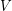be a vector space of dimensionand let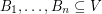be bases. Then there existdisjoint transversals ofeach of which is a base.

This very pretty conjecture of Rota has a number of interesting extensions. One (obvious) extension is to matroids, and indeed, Rota conjectured this generalization (just replace the vector spaceof dimensionby a matroid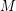of rank). This generalization has received some recent attention: Geelen and Humphries [GH] proved the special case whenis a paving matroid, Geelen and Webb [GW] proved that in general there existdisjoint transversals which are bases, and Aharoni and Berger [AB] used their beautiful "intersection of a matroid and a simplicial complex" theorem to prove that the union of the bases can be partitioned into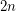partial independent transversals. Another generalization of Rota's basis conjecture is the following.

Conjecture  (Kahn)   Letbe a vector space of dimensionand for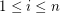and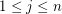let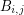be a basis. Then we may choose a vectorfrom each basisso that in the matrix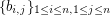, every row and every column is a basis.

To the best of my (M. DeVos') knowledge, this conjecture may generalize to matroids as well. There is another conjecture which implies Rota's basis conjecture which is far less obvious. In fact, Rota's conjecture (for evenand characteristic zero) is implied by Alon and Tarsi's Even vs. odd latin squares conjecture. This implication was first discovered by Rota and Huang [RH] (Rota had himself made another conjecture equivalent to that of Alon and Tarsi), and a very transparent proof of this fact based on a polynomial identity is given by Shmuel Onn [O].

Thanks to this last implication, partial results on the Alon-Tarsi conjecture show that Rota's basis conjecture holds wheneverhas the formor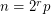whereis an odd prime [D1], [D2], [Z].

## Bibliography

[AB] R. Aharoni, E. Berger, The intersection of a matroid and a simplicial complex. Trans. Amer. Math. Soc. 358 (2006), no. 11, 4895--4917 MathSciNet.

[AT] N. Alon, M. Tarsi, Coloring and Orientations of Graphs. Combinatorica 12, 125-143, 1992 MathSciNet

[D1] A. Drisko, On the number of even and odd Latin squares of order, Adv. Math. 128 (1997), no. 1, 20--35. MathSciNet

[D2] A. Drisko, Proof of the Alon-Tarsi conjecture for. Electron. J. Combin. 5 (1998) MathSciNet.

[GH] J. Geelen, and P. J. Humphries, Rota's basis conjecture for paving matroids. SIAM J. Discrete Math. 20 (2006), no. 4, 1042--1045 MathSciNet.

[GW] J. Geelen, and K. Webb, On Rota's basis conjecture

*[HR] R. Huang and G-C Rota, On the relations of various conjectures on Latin squares and straightening coefficients. Discrete Math. 128 (1994), no. 1-3, 225--236. MathSciNet.

[O] S. Onn, A colorful determinantal identity, a conjecture of Rota, and Latin squares. Amer. Math. Monthly 104 (1997), no. 2, 156--159. MathSciNet.

[W] M. Wild, On Rota's problem aboutbases in a rankmatroid. Adv. Math. 108 (1994), no. 2, 336--345. MathSciNet.

[Z] P. Zappa, The Cayley determinant of the determinant tensor and the Alon-Tarsi Conjecture, Adv. Appl. Math. 19 (1997), 31--44.

* indicates original appearance(s) of problem.